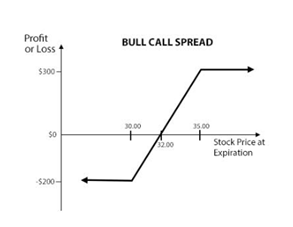Finschool By 5paisa

• #
• A
• B
• C
• D
• E
• F
• G
• H
• I
• J
• K
• L
• M
• N
• O
• P
• Q
• R
• S
• T
• U
• V
• W
• X
• Y
• ZA bull spread is an optimistic options strategy used when the investor expects a moderate rise in the price of the underlying asset. Bull spreads come in two types: bull call spreads, which use call options, and bull put spreads, which use put options. Bull spreads involve simultaneously buying and selling options with the same expiration date on the same asset, but at different strike prices. Bull spreads achieve maximum profit if the underlying asset closes at or above the higher strike price.

A bull call spread can be constructed by buying a call option with a lower strike price while simultaneously selling a call option with a higher strike price, on the same underlying security, expiring on the same date.Example

Suppose the XYZ stock is trading at Rs 32 and the option contract lot size is 100. A trader enters a bull call spread by buying ITM call at \$30 for Rs 300 and writing an OTM call at Rs 35 for Rs 100. The net investment required for the spread is Rs 200.

Suppose the stock price of XYZ begins to rise and closes at Rs 36 on the expiration date. Both options expire in-the-money, with the Rs 30 long call having an intrinsic value of \$600 and the Rs 35 short call having an intrinsic value of Rs 100. This means that the spread is now worth \$500 at expiration and the net profit is Rs 300.

If the price of XYZ had declined to Rs 29, both options expire worthless. The trader will lose his entire investment of Rs 200 which is also his maximum possible loss.

Bull put spread can be constructed by buying a put option with lower strike price and simultaneously selling higher strike put option on the same underlying stock, expiring on the same date.Example

Suppose the XYZ stock trading at Rs 33. A trader enters a bull put spread by buying OTM put at Rs 30 for Rs 100 and writing ITM put at Rs 35 for Rs 300. The trader receives a net credit of Rs 200 while entering the spread position.

Suppose the stock price of XYZ begins to rise and closes at Rs 36 on the expiration date. Both options expire worthless and the option trader keeps the entire credit of Rs 200 as profit, which is also the maximum profit possible.

If the price of XYZ is declined to Rs 29, both options expire in-the-money with the long call having an intrinsic value of Rs 100 and the short call having an intrinsic value of Rs 600. This means that the spread is now worth a negative Rs 500 at expiration. Since the trader had received a credit of Rs 200 when he entered the spread, his net loss comes to Rs 300. This is also his maximum possible loss.

View All